# Bihar Board Class 12 Chemistry Solutions Chapter 7 The p-Block Elements

Bihar Board Class 12 Chemistry Solutions Chapter 7 The p-Block Elements Textbook Questions and Answers, Additional Important Questions, Notes.

## BSEB Bihar Board Class 12 Chemistry Solutions Chapter 7 The p-Block Elements

### Bihar Board Class 12 Chemistry The p-Block Elements Intext Questions and Answers

Question 1.
Why are pentahalides more covalent than trihalides?
Higher the positive oxidation of central atom, [ + 5 and + 3 in pentahalides and trihalides respectively] more will be its polarising power which, in turn, increases the covalent character of bond formed between the central atom and the other atom.

Question 2.
Why is BiH3 the strongest reducing agent amongst all the hydrides of Group 15 elements?
BiH3 is the strongest reducing agent amongst all the hydrides Of Group 15, because it is the least stable out of all hydrides of Group 15 elements.

Question 3.
Why is N2 less reactive at room temperature?
The bond enthalpy of triple bond in N≡N is very high due to pπ-pπ overlap. Hence N2 is less reactive at room temperature. It reacts only at high temperature.

Question 4.
Mention the conditions required to maximise the yield of ammonia.
N2 (g) + 3H2 (g) → 12NH, (g); ΔfH° = – 46.1 kj mol-1
According to Le-Chatelier’s principle, high pressure (200 atm) and a temp, of ~ 700 K along with a catalyst of iron oxide with small amounts of K2O and Al2O3 are used to maximise yield of ammonia.

Question 5.
How does ammonia react with a solution of Cu2+?
Ammonia reacts with the blue coloured solution of Cu2+ ions it forms a deep-blue coloured complex according to the following reaction.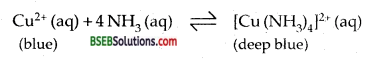Question 6.
What is the covalence of nitrogen in N2O5?
From the structure of N2O5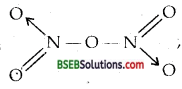It is evident that the covalence of N is four.

Question 7.
Bond angle in PH4+ is higher than that in PH3. Why?
P in both PH3, PH4+ is sp3 hybridized. In PH4+ ail the four orbitals are bonded, whereas in PH3, there is a lone pair of electrons on P. It is responsible for lone pair-bond pair repulsion in PH3 reducing the bond angle to less than the normal 109°28′.Question 8.
What happens when white phosphorus is heated with concentrated NaOH solution in an inert atmosphere of CO2?
When white phosphorus is heated with concentrated NaOH solution in an inert atmosphere of CO2 phosphine (PH3) is produced according to the following reaction.Question 9.
What happens when PCl5 is heated?
PCI5 sublimes on heating, but decomposes on further heating.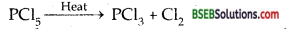Question 10.
Write a balanced equation for the hydrolytic reaction of PCl5 in heavy water.Question 11.
What is the basicity of H3PO4?
In the molecule of H3PO4, three P-OH groups are present.∴ Its basicity is three.

Question 12.
What happens when H3PO3 is heated?
H3PO3 on heating undergoes disproportionation to give phosphine (PH3) and orthophosphoric acid (or phosphoric acid)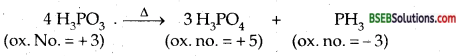Question 13.
List the important sources of sulphur.
The abundance of sulphur in the earth’s crust is only 0.03 – 0.1%. Combined Sulphur exists primarily as sulphates such as gypsum CaSO4. 2H2O Epsom salt MgSO4.7H2O, baryte (BaSO4) and sulphides such as galena (PbS), Zinc blende (ZnS), Copper pyrites (CuFeS2). Traces of sulphur occur as H2S in volcano, organic material such as eggs, proteins, garlic, onion, mustard, hair, and wool contain sulphur.

Question 14.
Write the order of thermal stability of the hydrides of Group 16 elements.
The thermal stability of the hydrides decreases in the order H2O > H2S > H2Se > H2Te > H2PO.Question 15.
Why is H2O a liquid and H2S a gas?
There is strong hydrogen bonding in H2O due to small size and high electronegativity of oxygen which is not present in H2S. Due to hydrogen bonding extra energy in the form of heat is required to break them. Hence H2O is a liquid at room temp.

Question 16.
Which of the following does not react with oxygen directly? Zn, Ti, Pt,Te.
Pt.

Question 17.
Complete the following reactions: .
(i) C2H4 + O2
(ii) 4 Al + 3O2
(i) C2H4 + 3O2→ 2 CO2 (g) + 2 H2O
(ii) 4 Al + 3O2 → 2 Al2O3.

Question 18.
Why does O3 act as a powerful oxidizing agent?
Due to the ease with which it can liberate atoms of nascent oxygen
O3 → O2 + O,
it acts as a powerful oxidizing agent.

Question 19.
How is O3 estimated quantitatively?
When ozone reacts with an excess of potassium iodide solution as.
2I (aq) + H2O (l) + O3 (g) → 2 OH (aq) +I2 (s) + O2 (g),
I2 is liberated which can be titrated against a standard solution of sodium thiosulphate (Na2S2O3). It is a quantitative method of estimating the O3 gas.

Question 20.
What happens when sulphur dioxide is passed through on aqueous solution of Fe (III) salt?
SO2, when passed through an aqueous solution of Fe (III) ions, reduces it to Fe (II) ions.
2 Fe3+ (aq) + SO2 (g) + 2 H2O (l) → 2 Fe2+ (aq) + SO42- (aq) + 4H+ (aq).Question 21.
Comment on the nature of two S-O bonds formed in SO2 molecule. Are the two S-O bonds in this molecule equal?
Both the S-O bonds in SO2 are covalent and have equal strength due to resonating/canonical structure.Question 22.
How is the presence of SO2 detected?
It is passed through an acidified solution of potassium permanganate which is rendered colourless by it.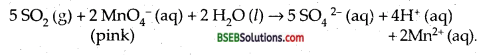Question 23.
Mention three areas in which H2SO4 plays an important role.

1. The bulk of H2SO4 is used in the manufacture of fertilizers like ammonia sulphate, superphosphate.
2. It is used as a dehydrating agent in the laboratory.
3. In tire manufacture of acids like HCl, HNO3 etc.

Question 24.
Write the conditions to maximise the yield of H2SO4 by Contact process.
Tire key reaction in the manufacture of H2SO4 by Contact process is the conversion of SO2 into SO3.The reaction is (i) reversibly, (ii) exothermic, (iii) proceeds with a decrease in voluble. Low temp, and high pressure are the favourable conditions for maximum yield. But the temperature should not be very low, other wise rate of reaction will become slow.
In practise the plant is operated at 720 K and a pressure of 2 bar. A catalyst of V2O5 is used to speed up the reaction.

Question 25.
Why is Ka2 < < Ka1 for H2SO4 in water?
HM2SO4 is a very strong acid in water largely because of its first ionisation to H3O+ and HSO4 The ionisation of HSO4 to H3O+ and SO42-is very small. That is why Ka2 < < Ka1.

Question 26.
Considering the parameters such as bond dissociation enthalpy, electron gain enthalpy and hydration enthalpy, compare the oxidising power of F2 and Cl2.
F2 is a stronger oxidizing agent than Cl2 due to

• low enthalpy of dissociation of F-F bond.
• high hydration enthalpy of F.

Question 27.
Give two examples to show the anomalous behaviour of fluorine.

1. Hydride of F (HF) is a liquid whereas HCl, HBr, HI are gases because HF shows hydrogen bonding.
2. F is the only halogen which shows an oxidation state of -1, whereas others show ox. states of +1, + 3, + 5, + 7 in addition to – 1.

Question 28.
Sea is the greatest source of some halogens. Comment.
Sea is the greatest source of halogens (meaning sea-salt producers) Seawater contains chlorides, bromides and iodides of sodium, potassium, magnesium and calcium, but is mainly sodium chloride solution (2.5% by mass). The deposit of dried up seas contain these compounds, e.g, sodium chloride and carnallite, KCl.MgCl2.6H2O. Certain forms of marine life contain iodine in their systems; various seaweeds, for example, contain upto 0.5% of iodine.

Question 29.
Give the reason for bleaching action of Cl2.
Chlorine when dissolved in water (moisture) releases nascent oxygen which is responsible for bleaching coloured matter due to oxidation.
H2O + Cl2 → HCl + HOCl (Hypochlorous acid)
HOCl → HCl + O (nascent oxygen)
Coloured substance + O → Colourless substance.Question 30.
Name two poisonous gases which can be prepared from chlorine gas. ,
Phosgene (COCl2), tear gas (CCl3NO2).

Question 31.
Why is ICI more reactive than I2?
I-Cl bond is weaker than I-I bond. Hence I-Cl is more reactive that I2.

Question 32.
Why is helium used in diving apparatus?
It is used as a diluent for oxygen in modem diving apparatus because of its very low solubility in blood.

Question 33.
Balance the following equation.
XeF6 + H2O → XeO2F2 + HF
XeF6 +2H2O→ XeO2F2 + 4HF.

Question 34.
Why has it been difficult to study the chemistry of radon?

### Bihar Board Class 12 Chemistry The p-Block Elements Text Book Questions and Answers

Question 1.
Discuss the general characteristics of Group 15 elements with reference to their electronic configuration, oxidation state, atomic size, ionisation enthalpy and electronegativity.
Electronic Configuration-All the elements of Group 15 have ns2np3 (= 5 electrons) electronic configuration in their valence shells. The s-subshell is completely filled and p-subshell is exactly half-filled
(p1xP1yP1z ) This imparts extra stability to their electronic configuration.
Nitrogen = N = 7 = [He] 2s22p3
Phosphorus = P = 15 = [Ne] 3s2 3p3
Arsenic = As = 33 = [Ar] 3d10 4s2 4p3
Antimony = Sb = 51 = [Kr] 4d105s2 5p3
Bismuth = Bi = 83 = [Xe] 4f145d10 6s26p3

Oxidation State-The common oxidation states of these elements are – 3, + 3, and + 5. The tendency to show – 3 oxidation state decreases from top to bottom due to increase in size and metallic character. N does not show + 5 oxidation state because it has only 4 orbitals (One s and three p) for bonding. Br hardly forms any compound in the – 3 oxidation state. The stability of + 5 oxidation state decreases down the group and that of + 3 state increases (due to inert-pair effect). N exhibits +1, + 2, and + 4 oxidation states, when it reacts with oxygen. P also shows + 1 and + 4 oxidation states in some oxoacids.

Atomic Size-Atomic size increases from N to Bi due to the addition of one orbit each time. There is a considerable increase in covalent radius from N to P. However from As to Bi; only a small increase in covalent radius is observed due to the presence of completely filled d and/or f orbitals in heavier members.

Ionisation Enthalpy-The ionisation enthalpy of Group 15 elements is much greater than that of Group 14 elements due to the extra stability of half-filled p-orbitals and smaller size. It decreases from top to bottom due to gradual increase in atomic size.
Electronegativity-Electronegativity decreases from top to bottom in the group. The differenced electronegativity is not much pronounced amongst the heavier elements.

Question 2.
Why does the reactivity of nitrogen differ from phosphorus?
The reactivity of Nitrogen differs from P due to its

• small size
• high ionisation enthalpy and electronegativity.
• non-availability of d-orbitals with N.
• Nitrogen has unique ability to form pn-pn multiple bonds
with itself and with other elements having small size and high electronegativity.

Question 3.
Discuss the trends in chemical reactivity of group 15 elements.
N differs from other members of the group in its chemical reactivity. Some important trends in the chemical reactivity of group 15 elements are as follows :
Hydrides-All the elements of Group 15 form volatile hydrides of the formula EH3 where E = N, P, As, Sb, Bi. The lighter elements also form hydrides of the formula E2H4. N forms HN3.

Oxides-N has a strong tendency to form pπ-pπ multiple bonding with N and O atoms, whereas other elements of this group do not. Actually, N forms five oxides with oxidation states ranging from +1 to + 5 [N2O, NO, N2O3, NO2/N2O4, N2O5 respectively] while other elements form oxides only in + 3 and + 5 oxidation states only [like P4O6 and P4O10]
Halides-The elements of Group 15 form halides of two types- Trihalides and Pentahalides: EX3 where E = N, P, As, Sb, Bi and X = F, Cl, Br and I. EX5 where E = P, As, Sb, Bi.Question 4.
Why does NH3 form hydrogen bond but PH3 does not?
N in ammonia forms hydrogen bond, but there are no hydrogen bonds present in PH3 due to

• N is small-sized as compared to P.
• N has higher value of electronegativity than that of P.

Question 5.
How is nitrogen prepared in the laboratory? Write the chemical equations of the reactions involved.
Laboratory method for the preparation of N2
(i) It is prepared by the reaction of ammonium chloride and sodium nitrite in the aqueous state. Small amounts of NO and HNO3 formed during the reaction can be removed by passing the gas through aq. H2SO4 containing K2Cr2O7.
NH4Cl (aq) + NaNO2 (aq) → N2 (g) + NaCl (aq) + 2H2O (l)
(ii) It can also be prepared from the thermal decomposition of ammonium dichromate: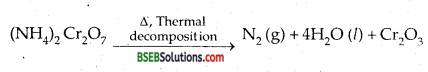(iii) Pure N2 can be prepared from sodium azide/Barium azide.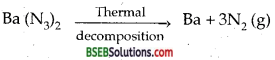Question 6.
How is ammonia manufactured industrially?
Ammonia is manufactured industrially by Haber’s Process
N2 (g) + 3H2 (g) → 2 NH3 (g); ΔH° = – 46.1 kj mol-1
In accordance with Le Chatelier’s principle, high pressure would favour the formation of ammonia. The optimum conditions for the ‘ production of ammonia are a pressure of 200 x 105 Pa (about 200 atm), a temperature of ~ 700 K and the use of a catalyst such as iron oxide with small amounts of KjO and A1203 to increase the rate of attainment of equilibrium. The flow chart for the production of ammonia is shown in figure below: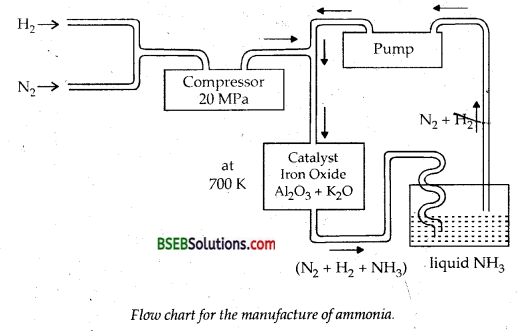Question 7.
Illustrate how copper metal can give different products on reaction with HNO3.
The products of oxidation of copper on reaction with HNO3 depend upon the concentration of the acid, and temperature of the reaction.
For example, Cu on reaction with dilute HNO3 gives the reaction.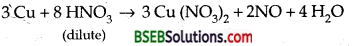and on reaction with concentrated HNO3, Cu gives the reaction.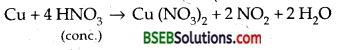Question 8.
Give the resonating structures of NOz and N2Os.
(i) NO2(ii) N2O5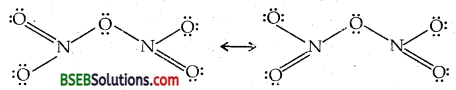Question 9.
The HNH angle value is higher than HPH, HAsH, HSbH angles. Why?
It can be explained on the basis of sp3 hybridisation in NH3 and only s-p bonding between hydrogen and other elements of the group: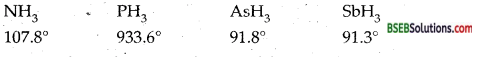As we move down the group, the size of the central atom goes on increasing and its electronegativity’ goes on decreasing. As a result, the bond pairs of electrons tend to lie away from the central atom as we move from NH3 to SbH3. In other words, the force of repulsion between the adjacent bond pairs is maximum in NH3 minimum in SbH3. Consequently, the bond angle is maximum in NH3 and minimum in SbH3.Question 10.
Why does R3P = O exist but R3N = 0 does not (R = alkyl group)?
Nitrogen due to lack of d-orbitals with it is not able to expand its covalency beyond 4 whereas P can. Hence R3N = 0 cannot exist whereas R3P = 0 can.

Question 11.
Explain why NH3 is basic while BiH3 is only feebly basic.
Due to the presence of lone pair of electrons on the central atom both NH3 and BiH3 are Lewis Bases. The decrease in basic character from NH3 to BiH3 can be explained in terms of electron density on the central atom. As the size of Bi atom is very large as compared to N, the lone pair of electrons occupies a larger volume, i.e., electron density on central atom decreases and consequently its tendency to donate a pair of electrons decreases and hence basic strength decreases in BiH3.

Question 12.
Nitrogen exists as diatomic molecule and phosphorus as P4. Why? –
Nitrogen because of its small size and high electronegativity forms pπ-pπ multiple bonds with itself. Thus nitrogen exists as triply bonded diatomic (N ≡ N) gaseous molecule at ordinary temperature. Phosphorus due to its larger size and lower electronegativity is reluctant to form pπ-pπ multiple bonds. Therefore, it prefers to form single bonds. Thus phosphorus exists as discrete tetra-atomic tetrahedral molecule as P4.

Question 13.
Write main differences between the properties of white phosphorus and red phosphorus.
Phosphorus exhibits allotropy. Tire most common allotrope is white phosphorous, which is formed by condensation from the gaseous or liquid states. It is a waxy solid, which is insoluble in water but highly soluble in CS2 and benzene. It is highly reactive and spontaneously ignites in air: Hence, it is stored underwater. It glows in dark and this property gives the element its name PHOSPHORUS (Greek for ‘light bringing’). white phosphorus consists of discrete P4 molecules in which the four phosphorus atoms are at the comers of a tetrahedron and each phosphorus atom is covalently linked to the other three phosphorus atoms. White phosphorous is highly toxic.

When white phosphorous is heated at 570 K in an inert atm asphere for several days, it gets converted into red phosphorous which has a higher melting point (870 K) and greater density (~ 2.16 g cm-3) than white phosphorus. Red phosphorous is amorphous and has a polymeric structure as shown (Fig.). Red phosphorous is much less reactive than white phosphorous and is safer and easier to handle. It is essentially non-toxic.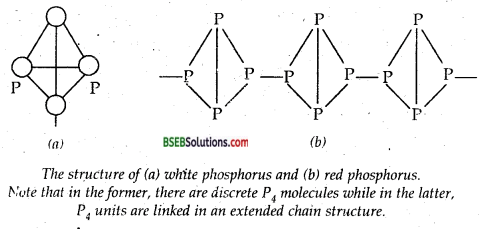Question 14.
Why does nitrogen show catenation properties less than phosphorus?
Due to smaller size, the lone pair of electrons on the N-atoms repel the bond pair of N-N bond. In contrast, because of comparatively. larger size of P, the lone pair of electrons or P atoms do not repel the bond pair of P-P bond to the same extent. As a result, N-N single bond is weaker than P-P, P-P single bond. Consequently, nitrogen shows little tendency for catenation while P has a distinct tendency for catenation.Question 15.
Give the disproportionation reaction of H3PO3.
Disproportionate reaction is one in which the same element undergoes both oxidation as well as reduction, i.e., there is an increase as well as decrease in its oxidation number. Thus + 3 oxidation state in H3PO3 increases to + 5 in H3PO4 and decreases to – 3 in the following reaction.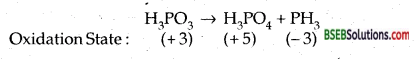Question 16.
Can PCl5 act as oxidising as well as a reducing agent? Justify.
Maximum oxidation state of P is + 5. P in PCl5 shows the maximum ox. state, i.e., + 5. If can decrease to + 3 and hence it can act as an oxidising agent.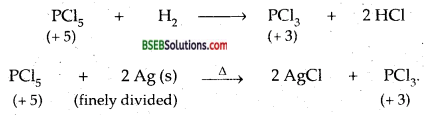As P in PCl5 is already in the maximum possible oxidation state (it has only 5 valence electrons in the stable electronic configuration of 3s23p5), Therefore it cannot increase its oxidation state and hence cannot act as a reducing agent.

Question 17.
Justify the placement of O, S, Se, Te and Po in the same group of the periodic table in terms of electronic configuration, oxidation state and hydride formation. „

• All the elements listed [O, S, Se, Te and Po] have a similar electronic configuration of ns2np4 (= 6 electrons in the valence shell), hence they are put in the same group.
• All these elements show oxidation states of-2 [except Po] + 2, + 4, + 6 [except O which shows only – 2, -1, + 1,
+2], Therefore, they have been put in the same group.
• All these elements of Group 16 form hydrides of the type H2E [E = 0,S, Se, Te, PoJ. All these hydrides except H2O possess reducing properties. Except H2O, all the hydrides are acidic in nature. These hydrides except water are thermally not stable.

Question 18.
Why is dioxygen a gas, but sulphur a solid?
Due to small size of oxygen, The non-bonding electrons on the two oxygen atoms in img bond strongly repel each other While such repulsions are much less in S-S bond. As a result, 0-0 bond dissociation energy is much less than that of S-S bond. In other words sulphur has much higher tendency for catenation and higher tendency for pji-pH multiple bonds (S does not form pπ-pπ S = S multiple bonds unlike 0 = 0 multiple bonds). Oxygen forms stable diatomic O2 molecule where as sulphur because of its higher .tendency for catenation and lesser tendency for pπ-pπ multiple bonds forms octa-atomic molecules having 8-membered puckered ring structure which exists as a solid.

Question 19.
Knowing the electron gain enthalpy values for O→ O and O → O2- as – 141 and 702 kj mol-1 respectively, how can you account for the formation of a large number of oxides having O2- species and not O?
Electron gain enthalpy values for O → O and O →O2-as – 141 and 702 kj mol-1 respectively, a large number of oxides having O2- species are formed instead of O because if we take into account lattice energy factor in the formation of O2 compound (in which large amount of energy in the form of lattice energy is released) overall enthalpy is negative.

Question 20.
Which aerosols deplete ozone?
Flurorochloro carbons or freons.

Question 21.
Describe the manufacture of H2SO4 by contact process.
Preparation of H2SO4 from by contact Process-
(i) SO2 is formed by burning Sulphur or roasting pyrites
S + O2 → SO2
4 FeS2 + 11 O2 → 2 Fe2O3 + 8 SO2

(ii) SO2 is oxidized to SO3 in the presence of V2O5 catalyst.(iii) Sulphur trioxide produced is absorbed in sulphuric acid to oleum which is further diluted with water to get sulphuric acid of desired concentration.
SO3 + H2SO4 → H2S2O7(Oleum),
H2S2O7 + H2O → 2H2SO4
Conditions for maximum yield-The reaction
2SO2 + O2 ⇌ 2SO3 +189 KJ
is reversible i.e., the sulphur trioxide formed by the oxidation of sulphur dioxide decomposes back to yield SO2 and O2 and an equilibrium is established In such reversible reaction, Le-chatter’s principle may be applied to get the maximum yield for sulphur trioxide.
(i) Air or excess of oxygen should be used.
(ii) Low temperature will favour the forward reaction because of its exothermic nature. But if the reaction is carried out at much low temperature, it becomes too slow. An optimum temperature of 720-770 K has been experimentally found for this reaction.

(iii) High pressure favours the forward reaction. But the reaction cannot be carried out at much higher pressures because acid-resisting
towers cannot be made to withstand high pressure. A pressure of two to three atmospheres is sufficient to make the steady flow of gases.

(iv) In the absence of a catalyst the reaction is too slow. A catalyst I increases the rate of oxidation of sulphur dioxide to sulphur trioxide. Previously, platinised asbestos was used as a catalyst. But it is very ; costly and also gets easily poisoned so that its efficiency decreases. Now a days, a cheaper catalyst vanadium pentoxide, V2O5, is used and it, j does not gets poisoned.

(v) As the catalyst is open to poisoning, the mixture of sulphur dioxide and air or oxgyen should be free from all types of impurities particularly the dust.

The plant and its working-The plant employed for the purpose and its working are as follows :
1. Sulphur or pyrite burners—Sulphur dioxide is obtained by : burning sulphur or iron pyrites in special type of burners made of
firebricks.
S + O2 → SO2
4 FeS2 + 11O2 → 2Fe2O3 + 8SO2

2. Purification unit-Sulphur dioxide obtained above contains sulphur or pyrites dust, arsenious oxide and sulphuric acid fog as the impurities. These, if not removed, would result in the poisoning of the catalyst. These are removed in the purification unit which consists of:
(i) Dust chamber-The mixture of gases is passed through a dust chamber from the top of which steam is allowed to come in. It makes the dust particles to settle down.

(ii) Cooling Pipes or Cooler-The gases coming out of the chamber are passed through a coded pipe. The temperature of the gases comes down to about 373 K. The pipes are cooled by air.

(iii) Water Scrubber-The gases from the cooling pipes are led into a tower called scrubber. It is packed with quartz. Water is sprayed from the top where it comes into contact with upgoing gases and washes the gases free from acid, mist and any soluble impurities.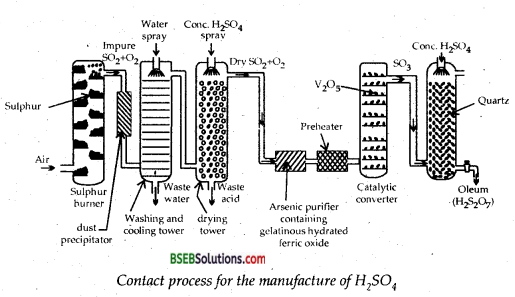(iv) Drying Tower-The outgoing gases from the scrubber are led into another tower which is packed with quartz. It is sprayed with concentrated sulphuric acid and the upgoing gases are freed from water because cone. H2SO4 acts as a dehydrating agent. Therefore, it is called drying tower.

(v) Arsenic-purifier-This is a small chamber fitted with shelves containing freshly precipitated ferric hydroxide. Here arsenic oxide present in the gases is absorbed by ferric hydroxide.

(vi) Testing Box-It is a rectangular chamber darkened from inside. A strong beam of light is passed in it and viewed through a hole perpendicular to the path of light. Solid particles present make the path of light visible due to the scattering of light by them (Tyndall Effect). Gases, if found free from the solid particles, are passed on the Contact Tower. If found to be impure, these are recycled through the purifying unit.

3. Contact Tower-The purified and dried gases are heated in a preheater and then passed through contact chamber. It is an iron chamber provided with iron tubes packed with platinised asbestos. More recently platinized asbestos has been replaced by more efficient catalyst vanadium pentoxide, V2O5. It is hot poisoned. The contact tower is heated to a temperature of about 720 K. Here SO2 combines with O2 of the air to form SO3.
2SO2 + O2 ⇌ 2SO3 + 189 kj
The reaction is exothermic and the heat evolved is used for heating the gases in the preheater.

4. Absorption Tower-SO3 from the contact tower is passed up an absorption tower where it meets a descending stream of concentrated (98%) sulphuric acid. This tower is also packed with quartz. SO3 is absorbed by H2SO4 when OLEUM is obtained,
SO3 + H2SO4 → H2S2O7 (Oleum)
To this oleum a calculated quantity of water is added to get the acid of desired concentration
H2S2O7 + H2O → 2H2SO4
Water is not used directly for dissolving SO3 because it forms a dense fog of sulphuric acid. It causes many difficulties  to the workers.Question 22.
How is SO2 an air pollutant?
SO2 dissolves in rainwater and produces acid rain.

Question 23.
Why are halogens strong oxidising agents?
Halogens act as strong oxidizing agents because they have strong tendency to accept electrons to complete their octets.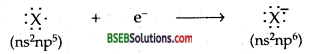Question 24.
Explain why fluorine forms only one oxoacid HOF?
Due to high electronegativity [F = 4.0, which is the highest in the periodic table] and smallest size amongst halogens it cannot act as central atom in higher oxoacids.

Question 25.
Explain why inspite of nearly the same electronegativity, oxygen forms hydrogen bonding while chlorine does not.
Oxygen which has only two orbits with it [1s2, 2s22p4] is smaller in size than Chlorine which has three orbits [1s2,2s22p6,3s23p5]. For hydrogen bonding the necessary condition is that the atom showing hydrogen bonding must be small in size. Smaller size favours hydrogen bonding. Hence O combined with H shows hydrogen bonding whereas Cl does not.

Question 26.
Write two uses of ClO2.

1. Chlorine dioxide (ClO2) is a powerful oxidizing agent.
2. It is a strong chlorinating agent and its bleaching power is about 30 times higher than that of Cl2.

Question 27.
Why are halogens coloured?
Halogen molecules absorb light in the visible region by means of which the outer electrons are excited to higher energy levels. The amount of energy required for the excitation (i.e., excitation energy) decreases progressively from fluorine to iodine as the size of the atom increases. Thus there is a progressive deepening of colour from F to I.

Question 28.
Write the reactidns of F2 and Cl2 with water.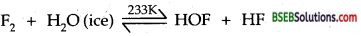HOF is unstable and decomposes of its own to HF and O2.
2 HOF → 2HF + O2
while chlorine dissolves in water to give the reaction.
Cl2 + H2O → HCl + HCIO Hypochlorous acid
HCIO → HCl + O Nascent Oxygen
The bleaching action of chlorine (moist) is due to nascent oxygen.

Question 29.
How can you prepare Cl2 from HCl and HCl from Cl2? Write reactions only.
(i) Cl2 from HCl
MnO2 + 4HCl → MnCl2 + Cl2 + 2H2O
2KMnO4 + 16 HCl→ 2 KCl + 2 MnCl2 + SCl2 + 8 H2OQuestion 30.
What inspired N. Bartlett for carrying out reaction between Xe and PtF6?
N Bartlett synthesised O2 PtF6 by the reaction between O2 and PtF6
O2 + PtF6 → O2+ [Pt F6]
Since oxygen and Xenon have nearly same ionisation enthalpies and their sizes are comparable. It prompted him to prepare from Xe and PtF6 the compound Xe+ [PtF6].
Xe (g) + PtF6 (g) → Xe+ [PtF6]Question 31.
What are the oxidation states of phosphorus in the following:
(i) H3PO3,
(ii) PCl3
(iii) Ca3P2,
(iv) Na3PO4,
(v) POF3.
(i) Let the Ox state of P be x in H3PO3,
3(+1) + x+3(-2) = 0
∴ +3+x-6 = 0
x = +3.

(ii) PCl3 :
x+3(-1) = 0
x =+3

(iii) Ca3P2:
3(+2)+2x = 0
2x = -6
x =-3.

(iv) Na3PO4:
3(+1)+x+4(-2)= 0
+3+x-8 = 0
x = +5

(v) POF3:
x +(-2)+3(-1) = 0
x -2-3 = 0
x = +5

Question 32.
Write balanced equations for the following:
(a) NaCl is heated with sulphuric acid in the presence of MnO2
(b) Chlorine gas is passed into a solution of Nal in water.
(a) MnO2 + 2 NaCl + 3 H2SO4 → 2NaHSO4 + MnSO4 + 2 H2O + Cl2
(b) Cl2 (g) + 2 Nal (aq) → 2 NaCl (aq) +I2

Question 33.
How are Xenon fluorides XeF2, XeF4 and XeF6 obtained?
(i) XeF2:(ii) XeF4:(iii)XeF6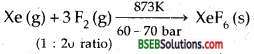(iv) XeF6 can also be prepared by the reaction between XeF4 and O2F2 at 143 K.
XeF4 + O2F2 → XeF6 + O2.

Question 34.
With what neutral molecule is CIO isoelectronic? Is that molecule a Lewis base?
(a) CIO is isoelectronic with ClF [Both have 26 electrons]
(b) Yes, ClF is a Lewis base because it can donate a lone pair of electrons.Question 35.
How are XeO3 and XeOF4 prepared?
(i) Slow hydrolysis of XeF6 by atmospheric moisture gives XeO3
XeP6 (s) + 3 H2O(l) → XeO3 (s) + 6 HF (aq) .
(ii) Partial hydrolysis of XeF6 gives XeOF4
XeF6 + H2O → XeOF4+2HF.

Question 36.
Arrange the following in the order of property indicated for each set:
(a) F2, Cl2, Br2, I2 – increasing bond dissociation enthalpy.
(b) HF, HCl, HBr, HI—increasing acid strength.
(c) NH3, PH3, AsH3, SbH3, BiH3-increasing base strength.
(a) I2 <F2< Br2 <Cl2
(b) HF < HCl < HBr < HI
(c) BiH3 < SbH3 < ASH3 < PH3 < NH3.

Question 37.
Which one of the following does not exist?
(a) XeOF4,
(b) NeF2,
(c) XeF2,
(d) XeF6.
(b) NeF2 does not exist.

Question 38.
Give the formula and describe the structure of a noble gas species which is isostructural with:
(a) ICl4
(b) IBr2
(c)BrO3
(a) XeF4 is isostructural with ICl4 Both are square planar.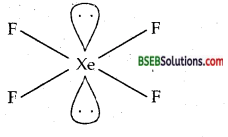(b) XeF2 is isostructural with IBr2 Both are linear.(c) XeO3 is isostructural with BrO3 Both are pyramidal.Question 39.
Why do noble gases have comparatively large atomic sizes?
Tire atomic sizes of noble gases are by far the largest in their respective periods. Noble gases do not form molecules and hence have only Van der Waals’ radii. Van der Waals’ radii by definition, are larger than covalent radii which the molecules usually have. As we move down, these atomic sizes further increase primarily due to the increase in the number of shells.

Question 40.
List the uses of neon and argon gases.
Uses of Neon
(i) Neon is mainly used in discharge tubes and fluorescent lamps for advertising purposes.
(ii) Neon has a remarkable property of carrying extremely high currents even under high voltage. Therefore, neon is used in safety devices for protecting electrical instruments like voltmeters, relays and rectifiers from high voltage.
(iii) It is used in becon light as safety signal for air navigators since its light has fog penetration power.

Uses of Argon-
(i) Argon is used mainly to provide inert atmosphere in high-temperature metallurgical processes such as are welding of metals and alloys. In the laboratory, it is used for handling air-sensitive substances.
(ii) It is used for filling incandescent and fluorescent lamps.
(iii) It is also used in neon signs for obtaining lights of different colours.

### Bihar Board Class 12 Chemistry The p-Block Elements Additional Important Questions and Answers

Question 1.
Write the structural formula of PCl5(s).
In the solid-state PCl5 exists as an ionic solid [PCl4]+ [PCl6] in which the cation is tetrahedral while the anion is octahedral.

Question 2.
Draw the structure of peroxo disulphuric acid.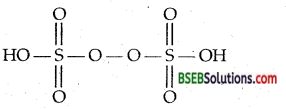Question 3.
Complete the following chemical equation. (AISB 2005)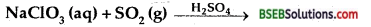Question 4.
Write the chemical equation for the following: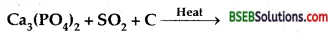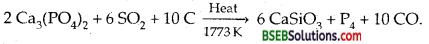Question 5.
Why do hydrides of oxygen and sulphuir differ in physical state? (P.S.B. 2005)
Hydrides of oxygen, i.e., H2O exists as an associate molecule (H2O)n due to intermolecular H-bonding and hence is a liquid at room temperature. Due to lack of H-bonding in the hydride of S (H2S) it exists of discrete molecules and hence is a gas at room temperature.

Question 6.
Write one chemical reaction to show that cone. H2SO4 can act as an oxidizing agent.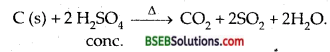Question 7.
Which is least basic? SbH3, PH3, NH3, AsH3? (H.S.B. 2005)
SbH3.Question 8.
Which halogen has highest electron affinity?
Chlorine (Cl).

Question 9.
Which type of hybridization explains that the shape of SF4 molecule is trigonal bipyramidal?
sp3d.

Question 10.
Which is more stable PCl5 or PCl3?
PCl3 is more stable. PCl5 on heating decomposes.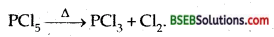Question 11.
Which is least acidic? SbH3, PH3, NH3, AsH3. (H.S.B. 2005)
A compound which is most basic is least acidic. Since NH3 is most basic and hence it is least acidic.

Question 12.
What type of hybridization is involved in PCl3?
sp3d.

Question 13.
Which hydride has greater bond angle H2O, H2S, H2Se, H2Te?
H2O.

Question 14.
Why is sulphurous acid a reducing agent?
Because it can be easily oxidized to H2SO4.
H2O + Br2 + H2SO3 → H2SO4+ 2 HBr.
∴ Sulphurous acid acts as a reducing agent.

Question 15.
The tendency to show – 2 oxidation state diminishes from sulphur to polonium. Why?
In Group 16, as we move down from sulphur to polonium, the electronegativity decreases.Question 16.
Why H2S is acidic while H2O is neutral?
The S-H bond in H2S is weaker than O-H “bond in H2O because’ the size of S-atom is larger than O-atom. Hence H2S dissociates in aqueous solution to give H+ ions.

Question 17.
Iodine is more soluble in KI than in water. Why? (P.S.B. 01,02, H.P.B.B. 97)
Iodine combines with KI to form soluble complex.
Kl+I2→ Kl3.

Question 18.
Why ammonia has higher boiling point than phosphine?
MH3 forms H-bonds but PH3 does not.

Question 19.
Name the three allotropes of phosphorus. Which of the three is most reactive? ‘
White/yellow, red and black. White phosphorus is most reactive.

Question 20.
Why does H3PO3 act as a reducing agent but H3PO4 does not?
P in H3PO3 is in + 3 oxidation state. It can extend its oxidation state to + 5. But the ox state of P in H3 Po4 is already in +5 oxidation state.

Question 21.
What is laughing gas? How is it prepared?
N2O. It is prepared by heating NH4NO3.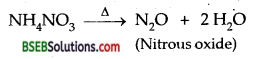Question 22.
Name the allotropes of oxygen.
Dioxygen (O2) and Ozone (O3).

Question 23.
Name the halogen which does not exhibit positive oxidation state.
Fluorine, because it is the most electronegative element.Question 24.
Give the shape of P4 and SO2.
P4 tetrahedral. SO2: angular with bond angle 119°.

Question 25.
Can FCl3 exist? Comment.
No, because F cannot exhibit valency more than one.

Question 26.
What is oleum? What is the oxidation state of Sulphur in it?
H2S2O7. Oxidation no. of S is + 6.

Question 27.
Why HI cannot be prepared by heating KI with cone.H2SO4?
HI initially formed being a strong reducing agent reduces H2SO4 to SO2 while itself is oxidized to I2.

Question 28.
Which of the following is/are not known?PCl3,AsCl3,SbCl3,NCl5,BiCl5,
NCl5 and BiCl5.

Question 29.
Why is HF weaker acid than HCl?
Due to stronger H-F bond than H-Cl bond, HF ionise to give H< sup>+ ions less readily than HCl in water.

Question 30.
Which one among the following is the strongest oxidizing agent? CIO4, BrO4,IO4
Amongst Cl4 (E° = +1.19 V), BrO4 (E° = +1.74 V), IO4 (E ° = +1.65 V) BrO4 has the highest reduction potential and hence it is the strongest oxidizing agent.Question 31.
What is the shape of XeF6 molecule? (H.P.S.B. 96)
Distorted octahedral.

Question 1.
Why is nitrous acid oxidant as well as reductant. (P.S.B. 2005)
The oxidation number of N in nitrous add (H-O-N = O) is + 3 which lies in between its lowest oxidation of – 3 and maximum oxidation state of + 5. Since Ox. no. of + 3 in HNO2 can both be decreased as well as increased. There it acts both as an oxidant and a reductant.

Question 2.
SiF62- is known but SiCl62- is not. Why is it so?
(i) Due to smaller size of F as compared to Cl, steric repulsions will be less in SiF62- than in SiCl62-.
(ii) Because of smaller Si-F bond as compared to Si-Cl bond the interaction of F lone pair of electrons with Si is stronger than that of Cl ions pairs.

Question 3.
Write the chemical equation to show that chlorine gas can be prepared from bleaching powder. (D.S.B. 2005)
Bleaching powder reacts with dilute acids like H2SO4, HCl etc. liberate Cl2 gas.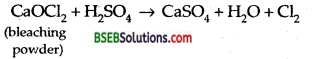Question 4.
Oxygen is diatomic and gaseous in nature, explain.
Due to small size and high electronegativity, oxygen forms pπ-pπ double bond and hence exists as diatomic molecules. Since these diatomic molecules are held together by weak Van der Waals forces of attraction which can be easily broken by collisions of the molecule at room temperature, therefore O2 is a gas.Question 5.
Give reasons for the least reactivity of nitrogen molecule.
Due to the presence of triple bond between the two nitrogen atoms (N ≡ N), tire bond dissociation energy of N2 is very high. Hence N2 is least reactive.

Question 6.
Why does argon not form diatomic molecules like oxygen and nitrogen?
All the orbitals which are occupied by electrons are completely filled in argon and hence it has no tendency to gain, lose, or share electrons with other atoms of Ar to form diatomic molecules. O and N, on the other hand, have impaired electrons and hence share with other O and N atom respectively to form O2 and N2 molecules.

Question 7.
How is pyrophosphoric acid related to orthophosphoric acid? Write its structure.
Pyrophosphoric acid (H4P2O7) is the anhydride of orthophosphoric acid and is obtained by removal of a molecule of H2O from two molecules of H3PO4. The structure of pyrophosphoric acid (H4P2O7)isQuestion 8.
Why is white phosphorus kept underwater? Name the three series of salts formed when orthophosphoric acid reacts with sodium hydroxide.

• Ignition temperature of white phosphorus is very low (303 K). Therefore, on exposure to air, it spontaneously catches fire forming P4O10.
• NaH2PO4, Na2HPO4, and Na3PO4.

Question 9.
Noble gases are mostly inert. Assign reasons. (D.S.B. 2005 S)
Noble gases are chemically inert because of the following reasons.

• They have completely filled ns2np6 electronic configuration in their valence shell [except He = 1s2]
• They have high ionisation enthalpies.
• Electron affinities of noble gases are almost zero.

Question 10.
Give reasons : (i) Xenon does not form fluorides such as XeF3 and XeF5,
(ii) Out of noble gases only Xenon is known to form established compounds.
(i) All the filled orbitals of Xenon have paired electrons. The promotion of one, two or three electrons from the 5p-filled orbitals to the 5d-vacant orbitals will give rise to two) four or six half-filled orbitals. So Xe can combine with even but not odd number of F atoms. Hence, it cannot form XeF3 and XeF5.

(ii) Except radon which is radioactive, Xe has least ionisation energy among noble gases and hence it readily forms chemical compounds, particularly with oxygen and fluorine.Question 11.
Why interh^alogens are more reactive than halogens?
This is because the bond in the interhalogen [A-X] is weaker than X-X bond in tire halogens. This is due to less effective overlapping or orbitals of dissimilar atoms than those between similar atoms.

Question 12.
Why HF is stored in wax-coated glass bottles?
This is because HF does not attack wax, but reacts with glass. It dissolves SiO2 present in glass-forming hydro Fluro silicic acid.
SiO2 + 6 HF → H2SiF6 + 2H2O.

Question 13.
Addition of Cl2 to KI solution gives it a brown colour but excess of Cl2 turns it colourless. Why? (D.S.B. 2005)
Cl2 being a stronger oxidizing agent than I2, just oxidizes KI to give I2 which imparts brown colour to the solution.
2 KI (aq) + Cl2 (g) → 2 KCl (aq) + I2 (s) (Brown)
If, however, Cl→ is passed in excess, the I2 formed gets further oxidized to iodic acid (HIO3) which is colourless.
5 Cl2 +I2 + 6H2O→10 HCl + 2 HIO3 (Colourless)

Question 14.
Why perchloric acid is stronger than H2SO4?
The ox. state of Cl in perchloric acid (HClO4) is + 7 while that of S in H2SO4 is + 6. As a result, ClO3 part of HClO4 can break the O-H bond more easily to librate a proton than H7SO4.

Question 15.
Basicity of H3PO2, H3PO3 and H3PO4 are same, justify or criticise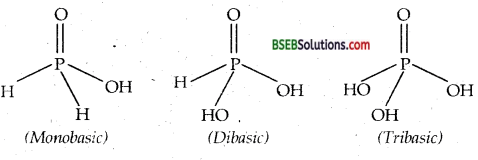Only OH group attached to P atom ionise to give H+ ions. Hence basicities are 1,2,3 respectively.

Question 1.
(a) How many grams of CaO are required to neutralise 852 g of P4O10? (b) Draw the structure of P4O10 molecules. (I.I.T 2005)
(c) Using VSEPR theory, draw the molecular structures of OSF4 and XeF4 indicating the location of lone pair(s) of electrons and hybridization of central atoms. (I.I.T. 2004)
(a) Tire chemical equation for the reaction is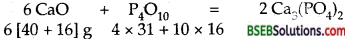288 g of P4O10 require CaO = 6 x 56 g
∴ 852 g of P4O10 will require CaO = $$\frac{6 \times 56}{288} \times 852$$ = 1008 g

(b) Structure of P4O10(c) (i) Structure of OSF4: In VSEPR theory, double bond is counted as one bond pair, therefore number of bond pairs around the central S atom.
= 4 × 1 (S-F) + 1 × 1 (S = 0) = 5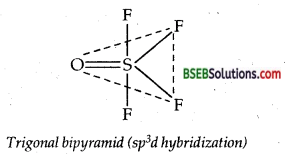No. of electron pairs around S =$$\frac{6+4+0}{2}$$ = 5
∴No. of lone pairs = 5-5 = 0
According’ to VSEPR theory, a molecule with 5 bond pairs and no lone pair must be trigonal bipyramid as shown in the figure.

(ii) Structure of XeF4
No. of bond pairs of electrons = 4
No. of lone pairs of electrons = 2
Total no. of electron pairs = sp3d2
Shape of XeF4 = Square planar